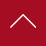• 年终奖
• 工作餐
• 公费旅游
• 公费培训
• 标准工作时间
• 带薪休

• 提成+奖金+生活补助
• 带薪休假
• 年轻化
• 团队氛围好
• 晋升空间大
• 工作氛围好

• 保险
• 年终奖
• 工作餐
• 公费旅游
• 公费培训
• 标准工作时间

• 带薪年假
• 五险
• 优秀员工奖
• 团队氛围好
• 晋升空间大
• 工作氛围好

• 保险
• 年终奖
• 公费旅游
• 公费培训
• 标准工作时间
• 带薪休假

• 保险
• 工作餐
• 公费旅游
• 公费培训
• 标准工作时间
• 带薪休假

• 保险
• 年终奖
• 工作餐
• 公费旅游
• 公费培训
• 标准工作时间

• 工作餐
• 公费旅游
• 公费培训
• 标准工作时间
• 带薪休

• 年终奖
• 公费旅游
• 公费培训
• 标准工作时间
• 带薪休

• 保险
• 工作餐
• 公费旅游
• 公费培训
• 标准工作时间

• 保险
• 年终奖
• 工作餐
• 公费旅游
• 公费培训
• 标准工作时间

• 保险
• 年终奖
• 公费旅游
• 公费培训
• 标准工作时间
• 带薪休假

• 提成，餐补，房补，话补，加班补助，年底双薪，环境好，免费培训

• 保险
• 年终奖
• 工作餐
• 公费旅游
• 公费培训
• 双休

• 年终奖
• 工作餐
• 公费培训
• 标准工作时

• 保险
• 工作餐
• 公费培训
• 标准工作时间

• 1
• 2
• 3
• 4
• 5
•••••